# Problem 59034. Compute the maximum length of a contaminant plume in groundwater

Problem statement
Write a function to compute the maximum length of a contaminant plume for steady, one-dimensional transport in groundwater. The leaking source has a constant concentration of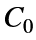, and the end of the plume for each contaminant is taken to be where the concentration equals some limit (e.g., the U.S. Environmental Protection Agency’s maximum contaminant level, or MCL). Assume the aquifer to be infinite and initially clean. Relevant properties of the confined aquifer are the hydraulic conductivity K, the magnitude of the head gradient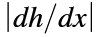, effective porosity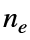, bulk density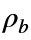, and soil organic fraction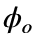. Along with the MCLs, the properties of the contaminants provided to the function are the half life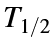and the octanol-water partition coefficient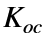.
Compute the longitudinal dispersion coefficient as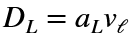, where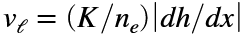is the average linear velocity and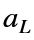is the longitudinal dispersivity. The variable aL will be specified as either a number or ‘X&E’. In the latter case, compute the longitudinal dispersivity with the empirical formula of Xu & Eckstein (1995):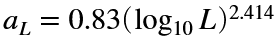whereis in meters if the flow length L is in meters. Take the flow length to be the length of the plume—that is, guess a plume length, compute the new plume, and repeat until the plume length stops changing.
Background
Contaminants in groundwater are subject to advection, retardation, dispersion, and degradation. If the transport is assumed to be one-dimensional, then the variation of the concentration C of the contaminant in time t and spatial coordinate x can be computed withThe four terms represent the effects of unsteadiness, advection, dispersion, and degradation, where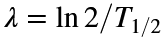. Retardation is included in the retardation coefficient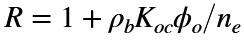. The maximum length occurs when all processes have had sufficient time to operate—that is, in steady state. In that case, the first term can be ignored, and the equation becomes a second-order linear ordinary differential equation with constant coefficients, which can be solved with the boundary conditions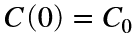and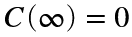.

### Solution Stats

100.0% Correct | 0.0% Incorrect
Last Solution submitted on Oct 03, 2023

### Community Treasure Hunt

Find the treasures in MATLAB Central and discover how the community can help you!

Start Hunting!